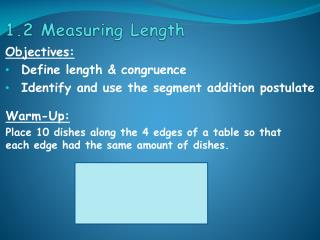DownloadDownload Presentation1.2 Measuring Length

# 1.2 Measuring Length

Télécharger la présentation## 1.2 Measuring Length

- - - - - - - - - - - - - - - - - - - - - - - - - - - E N D - - - - - - - - - - - - - - - - - - - - - - - - - - -
##### Presentation Transcript

1. 1.2 Measuring Length Warm-Up: Place 10 dishes along the 4 edges of a table so that each edge had the same amount of dishes. Objectives: Define length & congruence Identify and use the segment addition postulate

2. Vocabulary: Number Line: A line whose points correspond with the set of real numbers. -5 -4 -3 0 1 2 3 -2 -1 4 5

3. Vocabulary: Coordinate of a Point: The real number represented by a point on the number line. A -5 -4 -3 0 1 2 3 -2 -1 4 5 Note: 2 is the coordinate of point A

4. Vocabulary: Length of AB: The measure of AB is |a-b| or |b-a|. A B -5 -4 -3 0 1 2 3 -2 -1 4 5 AB = | a – b | = | -3 – 2| = | -5 | = 5 or AB = | b – a | = | 2 – -3| = | 2 + 3 | = | 5 | = 5

5. Vocabulary: Congruence: The relationship between figures have the same shape and same size. (congruent segments are segments that match exactly) ~ =

6. Vocabulary: Segment Congruence Postulate: If two segments have the same length then they are congruent. Also, if two segments are congruent they have the same length.

7. Vocabulary: Segment Addition Postulate: If point R is between points P and Q on a line, then PR + RQ = PQ P Q R RQ PR PQ

8. (The measure of AB is |a-b| or |b-a|.) Example 1: Find the measures (lengths) of AB, AX, & XB. A B X -5 -4 -3 0 1 2 3 -2 -1 4 5 AB = AX = XB =

9. Collins Writing Type 1: The length of a segment must be a positive number. Explain why the order of the coordinates does not matter when calculating length. _________________________________________ _________________________________________ _________________________________________ 3 lines ~ 2 minutes

10. Example 2: Name all of the congruent segments in each figure. A B E F C D H G AB, DC, EF, & HG and AD, BC, EH, & FG

11. Example 3: Point B is between points Q and R on QR. Sketch a figure for each set of values, and find the missing lengths. Q B R QB = 20; BR = 10; QR = ______. Q B R QB = 50; BR = ____; QR = 110. B Q R QB = ____ ; BR = 17; QR = 52.

12. Example 4: Find the indicated value. A B C AC = 60; x= _____. 4x 11x A B C AC = 36; x = ____. x 17

13. A B C 2x 4x+3 AB = 15; AC= _____. A B C x+2 3x+6 AC = 36; AB = ____.

14. Homework: Page 22 Numbers 11-25